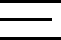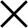Style

Main color

Motif

Weaving

Price

Finishing

Casual

€161.00

€156.00

€156.00

€156.00

€156.00

€181.00

€181.00

€181.00

€181.00

€176.00

€176.00

Blue Red Check Pattern Twill Shirt

High Amalfi Collar, Half-Moon Cuffs

€156.00
[profiler]
Memory usage: real: 47448064, emalloc: 46585576
Code ProfilerTimeCntEmallocRealMem
Executed 770 queries in 0.063775300979614 seconds
Average query length: 8.2825066207291E-5 seconds
Queries per second: 12073.639609261
Longest query length: 0.0026988983154297
Longest query:
SELECT `t_d`.`entity_id`, `t_d`.`attribute_id`, `t_d`.`value` AS `default_value`, `t_s`.`value` AS `store_value`, IF(t_s.value_id IS NULL, t_d.value, t_s.value) AS `value` FROM `catalog_product_entity_varchar` AS `t_d` LEFT JOIN `catalog_product_entity_varchar` AS `t_s` ON t_s.attribute_id = t_d.attribute_id AND t_s.entity_id = t_d.entity_id AND t_s.store_id = 2 WHERE (t_d.entity_type_id = 4) AND (t_d.entity_id IN (127355, 127354, 127225, 127224, 127223, 127415, 127352, 127351, 127349, 127356, 127361, 127350)) AND (t_d.attribute_id IN ('71', '86', '87', '97', '112', '113', '114', '118', '119', '213', '217', '218')) AND (t_d.store_id = 0) UNION ALL SELECT `t_d`.`entity_id`, `t_d`.`attribute_id`, `t_d`.`value` AS `default_value`, `t_s`.`value` AS `store_value`, IF(t_s.value_id IS NULL, t_d.value, t_s.value) AS `value` FROM `catalog_product_entity_text` AS `t_d` LEFT JOIN `catalog_product_entity_text` AS `t_s` ON t_s.attribute_id = t_d.attribute_id AND t_s.entity_id = t_d.entity_id AND t_s.store_id = 2 WHERE (t_d.entity_type_id = 4) AND (t_d.entity_id IN (127355, 127354, 127225, 127224, 127223, 127415, 127352, 127351, 127349, 127356, 127361, 127350)) AND (t_d.attribute_id IN ('73')) AND (t_d.store_id = 0) UNION ALL SELECT `t_d`.`entity_id`, `t_d`.`attribute_id`, `t_d`.`value` AS `default_value`, `t_s`.`value` AS `store_value`, IF(t_s.value_id IS NULL, t_d.value, t_s.value) AS `value` FROM `catalog_product_entity_decimal` AS `t_d` LEFT JOIN `catalog_product_entity_decimal` AS `t_s` ON t_s.attribute_id = t_d.attribute_id AND t_s.entity_id = t_d.entity_id AND t_s.store_id = 2 WHERE (t_d.entity_type_id = 4) AND (t_d.entity_id IN (127355, 127354, 127225, 127224, 127223, 127415, 127352, 127351, 127349, 127356, 127361, 127350)) AND (t_d.attribute_id IN ('75', '76', '120')) AND (t_d.store_id = 0) UNION ALL SELECT `t_d`.`entity_id`, `t_d`.`attribute_id`, `t_d`.`value` AS `default_value`, `t_s`.`value` AS `store_value`, IF(t_s.value_id IS NULL, t_d.value, t_s.value) AS `value` FROM `catalog_product_entity_datetime` AS `t_d` LEFT JOIN `catalog_product_entity_datetime` AS `t_s` ON t_s.attribute_id = t_d.attribute_id AND t_s.entity_id = t_d.entity_id AND t_s.store_id = 2 WHERE (t_d.entity_type_id = 4) AND (t_d.entity_id IN (127355, 127354, 127225, 127224, 127223, 127415, 127352, 127351, 127349, 127356, 127361, 127350)) AND (t_d.attribute_id IN ('77', '78', '93', '94')) AND (t_d.store_id = 0) UNION ALL SELECT `t_d`.`entity_id`, `t_d`.`attribute_id`, `t_d`.`value` AS `default_value`, `t_s`.`value` AS `store_value`, IF(t_s.value_id IS NULL, t_d.value, t_s.value) AS `value` FROM `catalog_product_entity_int` AS `t_d` LEFT JOIN `catalog_product_entity_int` AS `t_s` ON t_s.attribute_id = t_d.attribute_id AND t_s.entity_id = t_d.entity_id AND t_s.store_id = 2 WHERE (t_d.entity_type_id = 4) AND (t_d.entity_id IN (127355, 127354, 127225, 127224, 127223, 127415, 127352, 127351, 127349, 127356, 127361, 127350)) AND (t_d.attribute_id IN ('96', '122', '124', '126', '127', '128', '129', '132', '138', '165', '184', '224', '227')) AND (t_d.store_id = 0)